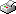Print This Page

Sofia Normark

-- licentiate thesis --

##### Topics in optimal design of experiments

Abstract
This thesis contributes to optimal design of experiments in situations where the optimal design depends on unknown parameters. Such situations often occur when the response variable does not have normal distribution, as is the case with many generalized linear models (GLM) and many models for binary response. In these cases the experimenter may either have to guess the values on the parameters and use a locally optimal design or use some method to ensure that the experiment will not yield parameter estimates with too large variance.

The thesis consists of two papers: In Paper I, optimal allocation to treatment groups for binary factorial experiments is studied. If the response variable is from the exponential family of distributions, and hence can be modeled by a GLM, the optimal allocation depends on the GLM weights, that in general are unknown. For this problem, a minimax design is considered. Under some conditions, the minimax allocation is as efficient as an optimal design. For other cases, no general conclusions can be drawn but an example suggests that the efficiency loss is small, compared to an optimal design.

Paper II examines the efficiency of locally optimal designs for a class of binary response models where the optimal design depends on values of unknown parameters. It is believed that if the parameter values that are used to construct the locally optimal design are close to the correct values, the resulting design may be "close to optimal". An approximation of the efficiency by a Taylor function is given. This function may be used to asses how far from the true values the guessed parameters may be, in order for the design to attain a minimum efficiency.

Keywords: optimal design, factorial experiments, minimax designs, efficiency, locally optimal designs, generalized linear models, binary data

ISBN: 978-91-7447-815-0